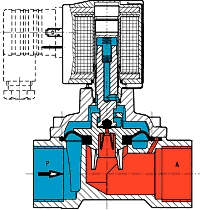# Kv Value Calculator - Liquids

This flow calculator is designed for calculating the required Kv flow requirements for use with liquids. Simply enter the details of your application and this results in the calculator giving you the required value in m3/h ready to select your Buschjost valve.

Flow Calculator - Liquids
GPM
Input required
kg/m3
Input required
barg
Input required
barg
Input required
Input required
0.00 bar
Calculation of Kv value
Calculation of Cv value
0.00 l/minThe calculation formulas are purely practical formulas and must only be used with the specified units. Please pay close attention to the specified units.

Δ p = p1 - p2

pü = p - pl

pü = Overpressure

p = absolute pressure of the medium

pl =Atmospheric pressure outside the valve

If the exact value of the atmospheric pressure (pl) is unknown, use ~ 1000 hPa (1 bar) as a default value.